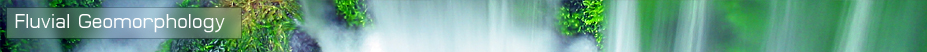Developed by T. Endreny at SUNY ESF in the Department of Environmental Resources Engineeringglossary | menu | Normal | lg | hc | 2.) FGM and Operational River Forecasting > Compute River Reach Sinuosity Exercise
 < Previous | 1 | 2 | 3 | 4 | 5 | 6 | 7 | 8 | 9 | 10 | 11 | 12 | 13 | 14 | 15 | 16 | 17 | 18 | 19 | 20 | 21 | 22 | 23 | 24 | Next >

# Compute River Reach Sinuosity Exercise### Exercise Objective

To learn how to compute South Brush River Reach Sinuosity using earlier measurements from topographic maps and possibly aerial photos.

### Exercise Process

This exercise requires prior completion of the two previous FGM exercises.
1. Compute river Reach Sinuosity, a measure of FGM pattern. There are two methods to compute Sinuosity, each explained below.
2. Use Valley and river Reach lengths to compute Sinuosity. Since the minimum value of Sinuosity a channel can have is 1, and given that between two river points, river Reaches often extend more distance than Valley distance, the Sinuosity can be computed as a quotient of river length and Valley length. Divide river length by Valley length and record the value of river Sinuosity.
3. Use Valley and river Reach slopes to compute Sinuosity. Divide the Valley slope by the Channel Slope. The logic of this quotient is based on Valley slopes being equal to or greater than the river, and hence in the numerator of the quotient to create a value of one or greater.

 < Previous | 1 | 2 | 3 | 4 | 5 | 6 | 7 | 8 | 9 | 10 | 11 | 12 | 13 | 14 | 15 | 16 | 17 | 18 | 19 | 20 | 21 | 22 | 23 | 24 | Next >